Question

Find an equation of the line​ L, where L is perpendicular to y = 2/3x and...

Find an equation of the line​ L, where L is perpendicular to y = 2/3x and passes through the point ​(3​,2​).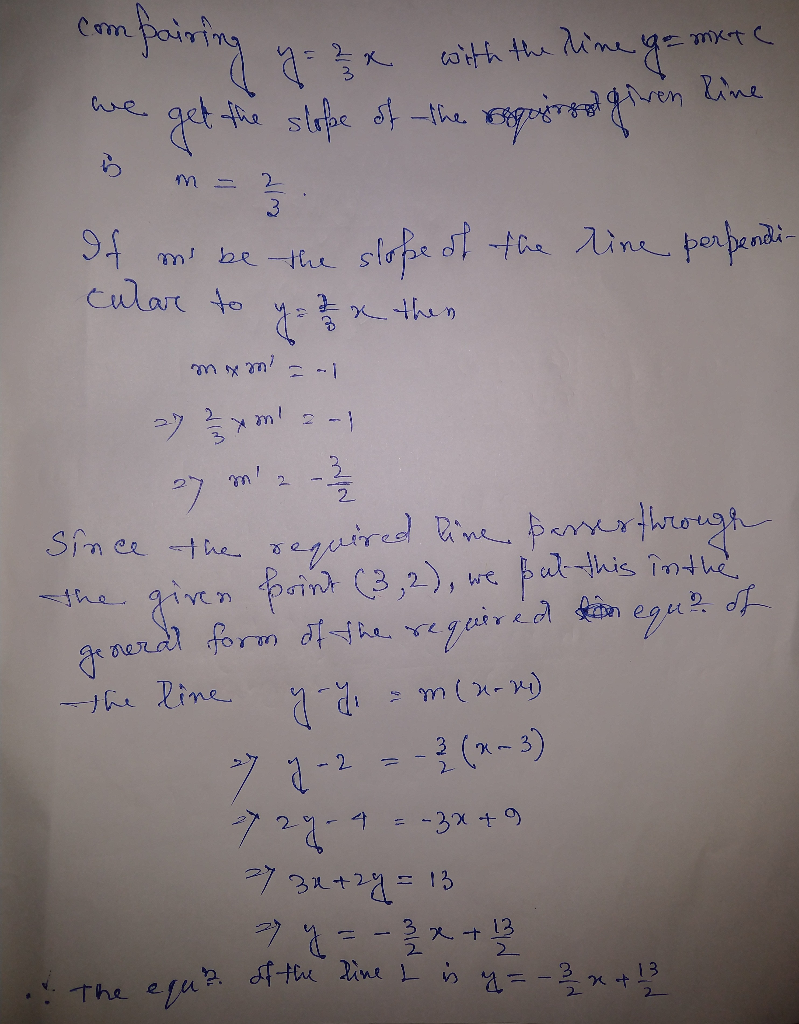Earn Coins

Coins can be redeemed for fabulous gifts.

Similar Homework Help Questions
• Find an equation that passes through (3,5) that is perpendicular to the line 3x+6y=4 equation that...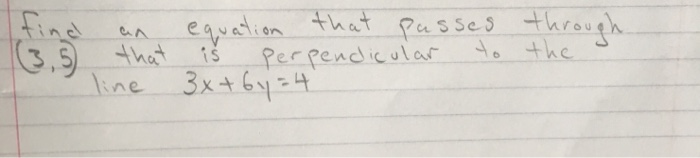Find an equation that passes through (3,5) that is perpendicular to the line 3x+6y=4 equation that passes passes through (3,5 that perpendicular to the line 3x+6y=4 is

• y^2-3x-4y-1=0 3. Find the equation of the tangent line and the equation of the perpendicular line...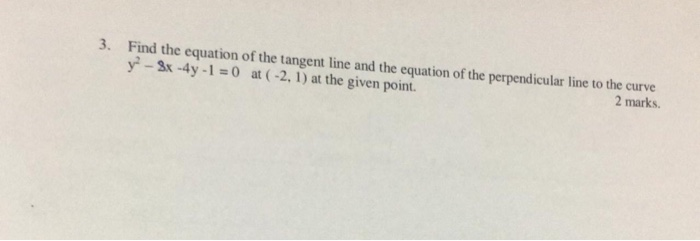y^2-3x-4y-1=0 3. Find the equation of the tangent line and the equation of the perpendicular line to the curve y? - 3x - 4y - 1 = 0 at (-2, 1) at the given point. 2 marks.

• Find the equation of the line satisfying the given conditions: perpendicular to the graph of y + 6 = -1 / 3x and passes through the point at (-4, -5)

Find the equation of the line satisfying the given conditions: perpendicular to the graph of y + 6 = -1 / 3x and passes through the point at (-4, -5). Find the equation of the line satisfying the given conditions: parallel to the x-axos and passes through the point (-5, -3). Use slope to show that (triangle symble)ABC with vertices A(0,2), B(2,3) and C(1,5) is a right triangle.

• Find the equation of the line perpendicular to 3x+y-2=0 that goes through the point, (0,1) Show...

Find the equation of the line perpendicular to 3x+y-2=0 that goes through the point, (0,1) Show all work.

• Write the standard form of the equation of the line that passes through (-3,7) and is perpendicular to the line with the equation y=3x-5

Write the standard form of the equation of the line that passes through (-3,7) and is perpendicular to the line with the equation y=3x-5.Answer: 3x+y=16Thanks

• Give the equation of the line that is perpendicular to the line y= -3x + 7 and passes through point (0, -6) please help

Give the equation of the line that is perpendicular to the line y= -3x + 7 and passes through point (0, -6)please help

• An equation of the line in slope intercept form that passes through the point (-9, -1) and is perpendicular to the line y = 3x + 17

An equation of the line in slope intercept form that passes through the point (-9, -1) and is perpendicular to the line y = 3x + 17?

• Find the equation of a line that is perpendicular to 3x-2y=6 and passes through (1,2)

Find the equation of a line that is perpendicular to 3x-2y=6 and passes through (1,2)

• Consider the line y=-5x+2. Find the equation of the line that is parallel to this line...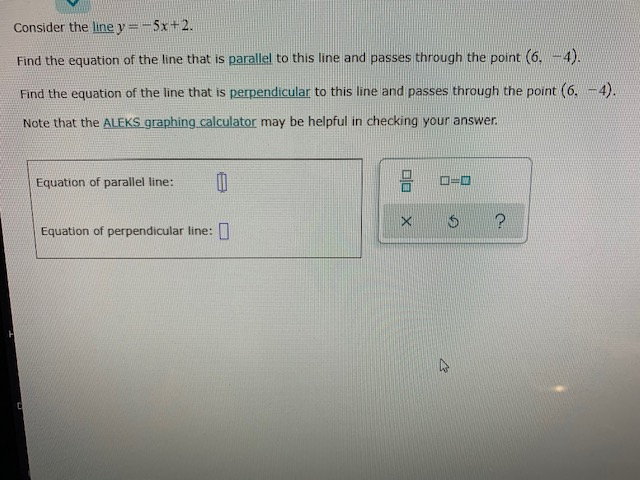Consider the line y=-5x+2. Find the equation of the line that is parallel to this line and passes through the point (6. 4). Find the equation of the line that is perpendicular to this line and passes through the point (6. -4). Note that the ALEKS graphing calculator may be helpful in checking your answer. E Equation of parallel line: DE X 5 ? Equation of perpendicular line:

• Parallel lines have the same slope. Perpendicular lines have negative-reciprocal slope. Find the equation of the...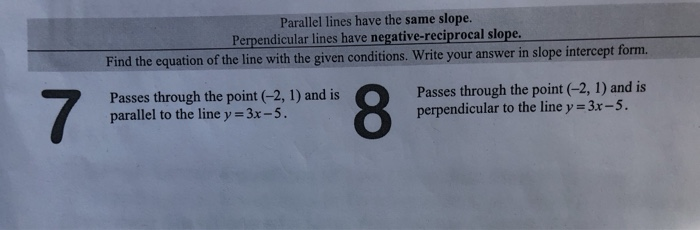Parallel lines have the same slope. Perpendicular lines have negative-reciprocal slope. Find the equation of the line with the given conditions. Write your answer in slope intercept form. Passes through the point (-2, 1) and is parallel to the line y = 3x -5. Passes through the point (-2, 1) and is perpendicular to the line y = 3x -5.